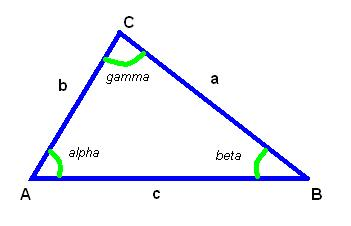# Triangle Angle Calculator

The Triangle Angle Calculator is a tool that calculates the angles of a triangle given the length of its sides.To use the calculator, the user simply inputs the length of the three sides of the triangle and the calculator will use the law of cosines to determine the angles. The results are displayed in degrees and can be used to solve problems in geometry, engineering, and other fields.

The law of cosines:
,
thus

For degenerate triangle, where side lengths violate the following inequalities
,
,
,
the results will be zeroes.#### Triangle angles for given triangle sides

Digits after the decimal point: 2
Angle alpha (degrees)

Angle beta (degrees)

Angle gamma (degrees)

URL copied to clipboard

#### Similar calculators

PLANETCALC, Triangle Angle Calculator